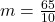## A body weighs 65N.Calculate it’s mass.(g=10N/kg)

Question

A body weighs 65N.Calculate it’s mass.(g=10N/kg)

in progress 0
2 months 2021-07-23T00:57:28+00:00 1 Answers 2 views 0

1. Explanation:

Hey there!!

Here,

Weighs = 65n

Gravity = 10n

We know that,

weighs = mass × gravity.

65 = m × 10Therefore the mass is 6.5 kg.

Hope it helps...